Next: A Polarization Model for Up: Introduction Previous: Resonant Depolarization   Contents

## Beam Excitation and Resonances

As already mentioned in chapter 2.3 there is a relationship between spin motion and orbital motion; this shall be examined in this section. Assume an electron performing vertical betatron oscillations according to Hill's Equation and consider these oscillations damped weakly: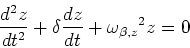(26)

where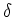is the damping constant and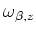is the vertical betatron oscillation frequency. Now a vertical kicker magnet shall be used to excite the beam. Compared to the perturbation of the beam through the kicker magnet damping is negligible: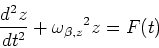(27)

The radial field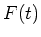is time dependent, but it is more complicated than simply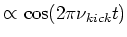because the electron only sees the excitation during the small period of time in which it travels through the kicker magnet. Thereforecan be arranged as a cosine multiplied with a step function :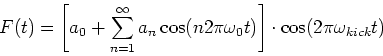(28)

where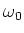is the revolution frequency. The solution to equation 27 is: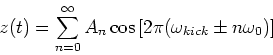(29)

and the amplitudes are determined by: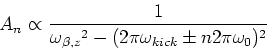(30)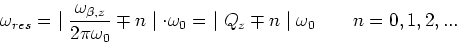(31)

(where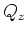is the vertical tune) which means that if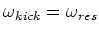the vertical beam oscillations will be excited to a point where the beam is lost. The same can be shown for the horizontal betatron oscillations (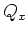) as well as for synchrotron motion (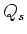). Such resonances must be avoided during the whole experiment.

The motion of the spin vector can be described in a very similar way: A solution to the Thomas-BMT equation (equation 11) is given by (neglecting phase)5: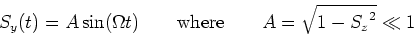(32)

Now the spin precession is excited with the kicker magnet: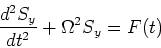(33)

Taking into account again thatis not a pure cosine, but a cosine multiplied with a step function, one derives: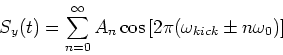(34)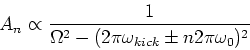(35)

Keeping in mind equations 11, 12 and 14 an recalling that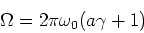(36)

the resonant depolarizing frequencies are derived: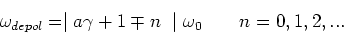(37)

This shows that the resonant depolarizer frequencies are sidebands of the revolution frequency. The case where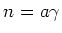is called the integer spin tune. If the kicker frequency is in resonance with the depolarizing frequency (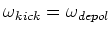) it is possible to tilt the mean spin vector of the electrons in the beam into the horizontal plane; in presence of spin diffusion effects (with a decoherence time much smaller than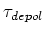) this depolarizes the beam.Next: A Polarization Model for Up: Introduction Previous: Resonant Depolarization   Contents
Simon Leemann
2002-03-15